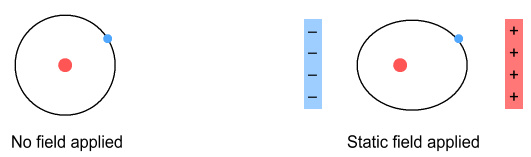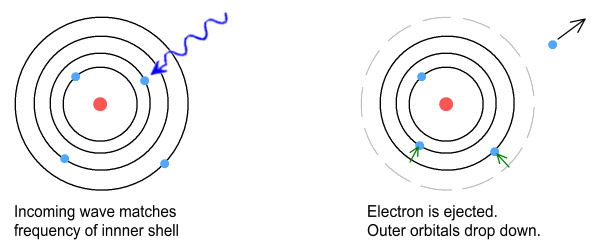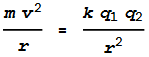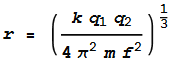Home

# The Photoelectric Effect

When light hits a metallic surface under suitable conditions we can observe electrons being ejected.   This is called the Photoelectric Effect and was first studied by Hertz and J.J. Thompson in the late 1800s.

The fact that this happens this should probably come as no surprise.  Light carries ‘energy’, which is to say it can cause electrons to move about and a surface to heat up.  Presumably then, it should occasionally cause electrons to be ejected altogether.

A closer examination into the effect however reveals some unexpected behaviour:

• Increasing the intensity of the light increases the number of ejected electrons per second, but their ejection speed (kinetic energy) remains the same.
• Light below a certain frequency ejects no electrons at all.  Whereas light above that threshold frequency can eject electrons, even when the light’s intensity is low.
• The ejection speed of electrons appears to be a function of frequency, such that an increase in frequency brings about an increase in speed.
• This threshold frequency is different for different materials, and different materials have different ejection speeds.
• The effect is seemingly immediate and doesn’t require the energy to be built-up over a period.

In response to this, and building on the work of Plank, Einstein postulated that light consists of discrete units, or ‘quanta’ of energy.  These units were later called photons, and the energy of each photon was said to be in direct proportion to its underlying frequency.   So in order to remove an election from an atom, i.e. to ionize it, the frequency of the photon must first be above the atom’s ionizing energy.  Otherwise the electron will stay put, regardless of how many photons rain down on it.

Under this proposal light took on a split personality; behaving sometimes as waves and other times as particles, depending on the experimental setup.  For example when producing interference patterns, light is considered a wave.  But for the Photoelectric effect and a related one called Compton scattering, it is considered a particle.  The contradictory nature of this proposal led to an ongoing debate over light’s dual wave/particle nature that continues to this day.

#### Another approach?

There is however a much simpler approach to explain what’s happening here, without having to postulate that light behaves both as a wave and a particle.  In fact when properly considered, what is observed is what we should logically expect from the classical orbital model of an atom.

Before getting to the details on this, it should be pointed out that the claim that low-frequency light can’t ionize an atom is incorrect.  One method of ionizing atoms is to expose them to a powerful electric field.  This can be done for example by applying a high voltage across opposing metal plates, giving rise to a static electric field between them.  Now technically speaking, a static (fixed) electric field is light of zero frequency.  Presumably this means that field has zero energy and nothing should happen.  But with enough voltage, electrons on the negative plate will be removed and jump across to the positive plate.  If done in air, this will result in a spark.

Another example would be the giant CO2 lasers used in an unsuccessful attempt to produce hot fusion.  These lasers use frequencies in the infrared range, which is much lower than what the photoelectric effect would require (usually ultraviolet).  And yet these intense low frequencies can ionize a substance into plasma, completely removing all of its electrons.

So clearly, given enough intensity, low or zero frequency light can remove electrons.

But putting aside situations involving ultra-intense amplitudes, it is true to say that frequencies below a certain level will be unable to remove electrons above a certain ‘energy level’.

#### The opera singer

To understand this, consider a tale that is sometimes told of an opera singer and a wine glass.  As she sings, the glass vibrates.  Then, just as she hits the right note, the glass vibrates so much it shatters.  This feat has been demonstrated by a number of singers, although is much easier to do with electronic sounds and amplifiers.

How does it happen?  The process is called ‘resonance’ and is due to ‘natural frequencies’.  All objects vibrate when exposed to sound.  And they do so more strongly when the impacting frequency corresponds to a natural vibrational frequency of the object in question.

Another example would be a piano.  When a separate instrument plays a note, the piano string corresponding to that note is observed to vibrate.  This is called ‘sympathetic vibration’ and is also due to natural frequencies.

#### The frequency of planets

Now consider the Solar System.  Our Sun is surrounded by a number of planets.  Each planet orbits in a roughly circular path, and with different orbital period.  The closest planet, Mercury, orbits once every 32 days.  While the furthest planet, Neptune (as now defined), does so once every 273 years.

Instead of describing planets according to orbital periods, we could do so in terms of frequency.  For example we could specify how many times they orbit the Sun every 1000 years.

Under this description, Mercury would have a frequency of 11,400 (orbits per 1000 years), Earth would have exactly 1000, and Neptune’s frequency would be 3.67.  So the inner planets have the highest frequencies, and the outermost the lowest.

#### Orbital frequencies of electrons

This planetary model is rather similar to the early Bohr model of the atom.  In Bohr’s model, electrons circle the nucleus at differing distances.  The inner electrons orbit at high frequencies, and the outer electrons at lower frequencies.

Now for an important question:  What would happen if we exposed that atom to an electromagnetic wave who’s frequency matched the orbital frequency of one of its electrons?

Let’s first consider the impact of a fixed electric field.  That is, light of zero frequency.  What will happen?  If the field is weak, the atom’s (positive) nucleus will be pulled in one direction, and it’s electrons in the other.  The result would be an electron in an elliptic orbit.If the applied field was strong enough, it would pull the electron completely away, ionizing the atom.

Now consider the impact of a low-frequency wave, i.e. light with frequency lower than the electron’s orbital frequency.  As it impacts, the wave pushes the electron ‘to-and-fro’: sometimes in the same direction as what the electron is moving, and other times in the opposing direction.  Or if you prefer, sometimes pushing it toward the nucleus and other times away.

As a result the orbit is shaken up but not enough for it to break.  Once the incoming wave stops, the orbit returns to its previous circular path.

Now consider the situation where the input frequency matches the orbital frequency.  Initially there might be some ‘confusion’ as the incoming wave pushes and pulls in the opposing direction to what the electron is moving.  But after a short while the orbit will ‘phase lock’ or synchronise with the incoming wave.

The situation is now somewhat different from the preceding example.  Here the incoming wave pulls on the electron in an outward direction (away from the nucleus) during both ‘halves’ of the orbit.

The incoming wave in now at the ‘natural frequency’ of the electron’s motion.  Just like the wine glass, the orbit experiences so much force it is ‘shattered’.  Or rather, the electron is pulled away from the nucleus where the attractive force is reduced.

At this point the existing speed of the electron takes over and enables the electron to fly fully away from the nucleus.  The atom is ionized.  From an observer’s viewpoint it might appear that the incoming wave ionized the atom because the light’s frequency had the right ‘energy’.  But in reality it did so because it matched the frequency of the orbit.

The above describes a situation in which an electron can be ejected when the incoming wave matches the frequency of the orbit.  So what about the situation where the wave frequency is higher than the orbit frequency?  In this case the wave is no longer at the natural frequency and thus shouldn’t be able to eject the electron.  Except we know it does.

To understand why, look again at the Solar System.  Here we have many planets orbiting at different distances from the Sun.  The inner planets have the highest frequency and the outer planets the lowest.

An atom is a similar situation.  There are inner electrons orbiting at high frequency and outer ones at low frequency.  When a high frequency wave comes in, the outer electrons might not be very affected.  But the inner ones will be.  When an incoming wave matches the natural frequency of an inner electron, that electron will be ejected.

So how does this affect the outer electrons?  There are two possibilities to consider.  The first is that, in the process of being ejected, the inner electrons would pass through the orbits of the outer electrons.  This might destabilise their orbits to the point that they also got ejected.

The second and more likely scenario is that once the inner electrons got ejected, the outer electrons would drop in altitude to fill the newly vacated orbit space.  Once there, the electron that took up the old orbit would have the frequency of the incoming wave.  It too would be ejected and another electron would drop to fill that vacancy.  This process would continue until electrons of (originally) lower frequency (and higher altitude) were also ejected.Thus a high frequency corresponding to an electron in an inner orbit ends up ejecting all the electrons from the outer orbits.

Another thing that should be apparent is that higher frequencies correspond to higher orbit speeds.   And so an electron ejected from a higher orbit speed should be expected to leave the material surface faster.  This is consistent with the observation that higher frequencies eject electrons at higher energies (speeds).

It should also be noted that this ejection process would be quite fast.  I.e. it should happen within a few cycles of the incoming wave.  So to us it would appear instantaneous.  This is consistent with the observation that the photoelectric effect is immediate and doesn’t require a build-up of energy before an electron is ejected.

#### A simple test

In order to test the validity of this theory we need to ‘plug some numbers in’ to see how it matches the real world.  We can look at oxygen as an example.  Oxygen is ionized with ultraviolet light of frequency of 3.3x1015 Hz (or wavelength 91nm) .  What electron orbital radius does that correspond to?

Oxygen has 8 protons in the nucleus and as many electrons on the outside.  So each electron could be considered as orbiting a single proton because the positive field from the other 7 protons would be effectively cancelled by the negative field of other 7 electrons.  This leaves us with a very simple model of an electron orbiting a proton.  So the question is, how far from the proton would an electron need to be to orbit 3.3x1015 times a second?

To do this we need to find out when the outward centrifugal force matches the inward Coulomb force.  This will happen when:The left side of the equation is the centrifugal and the right side is the Coulomb.  We can substitute velocity v with v=2 pi r f, where f is the orbit frequency.  Isolating the radius term gives:Substituting in values: k=9x109, q1=q2=1.6x10-19 C, m=9.1x10-31 kg, f=3.3x1015, gives:

r= 8.4x10-11m or 84pm

Oxygen has a ‘Van der Waals’ radius of 152pm.  These numbers are very close – arguably too close to be put down to coincidence.  It thus shows that the explanation given here for the photoelectric effect is likely to be correct.  The difference between these numbers can be attributed to (a) the orbit model being simplistic and (b) the Van der Waals radius being an approximation of the outer orbit shell size.  For a closer match we could also look at oxygen’s covalent radius, which is listed as being 66pm.

#### Related effects

Finally, it should be mentioned that the ionizing process being described here doesn’t just apply to the photoelectric effect, i.e. where electrons are ejected from metals, but also to light-induced chemical reactions.

For example, exposing skin to ultraviolet light can cause sunburn.   But strong infrared light will cook you rather than give a tan.  Actually it needs to be a high-frequency ultraviolet because a UV ‘black light’ won’t cause sunburn either.  This tells us the frequency needs to be above a certain critical point for skin to be damaged in a manner consistent with sunburn.  This frequency probably corresponds to outer-shell electrons of similar frequency being removed, causing chemical bonds to be broken.

Another example would be photosynthesis, in which plants use ultraviolet light to convert carbon dioxide and water into sugar.  Apparently light in the low-frequency visible portion of the spectrum is unsuitable for the task, even when intense.  Again, this is likely due to electrons in the outer orbits of carbon and oxygen having a frequency in the ultraviolet range, and the CO2 bonds won’t be broken until certain outer-shell electrons are removed.

 http://en.wikipedia.org/wiki/List_of_fusion_experiments
 The ionizing energy of oxygen’s outer shell is 1313.9 kJ/mol.  Multiplying by 1000 and dividing by Avogadro’s constant gives us 2.18x10-18 J.  Dividing this by Plank’s constant gives a frequency of 3.29x1015 Hz.

 Previous Contents Next chapter